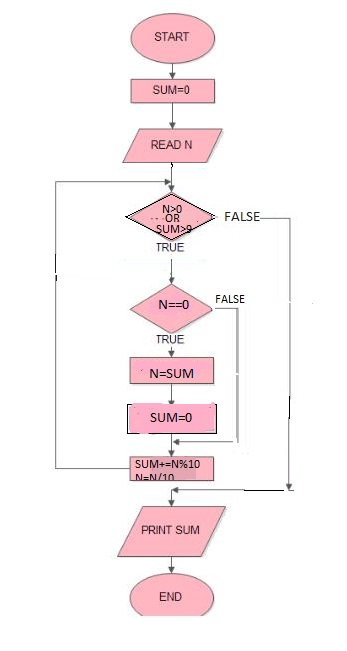Related Articles

# Finding sum of digits of a number until sum becomes single digit

• Difficulty Level : Medium
• Last Updated : 26 Mar, 2021

Given a number n, we need to find the sum of its digits such that:

```If n < 10
digSum(n) = n
Else
digSum(n) = Sum(digSum(n))```

Examples :

```Input : 1234
Output : 1
Explanation : The sum of 1+2+3+4 = 10,
digSum(x) == 10
Hence ans will be 1+0 = 1

Input : 5674
Output : 4 ```

A brute force approach is to sum all the digits until sum < 10.
Flowchart:Below is the brute force program to find the sum.

## C++

 `// C++ program to find sum of``// digits of a number until``// sum becomes single digit.``#include`` ` `using` `namespace` `std;` `int` `digSum(``int` `n)``{``    ``int` `sum = 0;``   ` `    ``// Loop to do sum while``    ``// sum is not less than``    ``// or equal to 9``    ``while``(n > 0 || sum > 9)``    ``{``        ``if``(n == 0)``        ``{``            ``n = sum;``            ``sum = 0;``        ``}``        ``sum += n % 10;``        ``n /= 10;``    ``}``    ``return` `sum;``}` `// Driver program to test the above function``int` `main()``{``    ``int` `n = 1234;``    ``cout << digSum(n);``    ``return` `0;``}`

## Java

 `// Java program to find sum of``// digits of a number until``// sum becomes single digit.``import` `java.util.*;` `public` `class` `GfG {``    ` `    ``static` `int` `digSum(``int` `n)``    ``{``        ``int` `sum = ``0``;` `        ``// Loop to do sum while``        ``// sum is not less than``        ``// or equal to 9``        ``while` `(n > ``0` `|| sum > ``9``)``        ``{``            ``if` `(n == ``0``) {``                ``n = sum;``                ``sum = ``0``;``            ``}``            ``sum += n % ``10``;``            ``n /= ``10``;``        ``}``        ``return` `sum;``    ``}``    ` `    ``// Driver code``    ``public` `static` `void` `main(String argc[])``    ``{``        ``int` `n = ``1234``;``        ``System.out.println(digSum(n));``    ``}``}` `// This code is contributed by Gitanjali.`

## Python

 `# Python program to find sum of``# digits of a number until``# sum becomes single digit.``import` `math` `# method to find sum of digits``# of a number until sum becomes``# single digit``def` `digSum( n):``    ``sum` `=` `0``    ` `    ``while``(n > ``0` `or` `sum` `> ``9``):``    ` `        ``if``(n ``=``=` `0``):``            ``n ``=` `sum``            ``sum` `=` `0``        ` `        ``sum` `+``=` `n ``%` `10``        ``n ``/``=` `10``    ` `    ``return` `sum` `# Driver method``n ``=` `1234``print` `(digSum(n))` `# This code is contributed by Gitanjali.`

## C#

 `// C# program to find sum of``// digits of a number until``// sum becomes single digit.``using` `System;` `class` `GFG {``    ` `    ``static` `int` `digSum(``int` `n)``    ``{``        ``int` `sum = 0;` `        ``// Loop to do sum while``        ``// sum is not less than``        ``// or equal to 9``        ``while` `(n > 0 || sum > 9)``        ``{``            ``if` `(n == 0)``            ``{``                ``n = sum;``                ``sum = 0;``            ``}``            ``sum += n % 10;``            ``n /= 10;``        ``}``        ``return` `sum;``    ``}``    ` `    ``// Driver code``    ``public` `static` `void` `Main()``    ``{``        ``int` `n = 1234;``        ``Console.Write(digSum(n));``    ``}``}` `// This code is contributed by nitin mittal`

## PHP

 ` 0 || ``\$sum` `> 9)``    ``{``        ``if``(``\$n` `== 0)``        ``{``            ``\$n` `= ``\$sum``;``            ``\$sum` `= 0;``        ``}``        ``\$sum` `+= ``\$n` `% 10;``        ``\$n` `= (int)``\$n` `/ 10;``    ``}``    ``return` `\$sum``;``}` `// Driver Code``\$n` `= 1234;``echo` `digSum(``\$n``);` `// This code is contributed``// by aj_36``?>`

## Javascript

 ``

Output :

`1`

There exists a simple and elegant O(1) solution for this too. The ans is given by simply :-

```If n == 0
return 0;

If n % 9 == 0
digSum(n) = 9
Else
digSum(n) = n % 9 ```

How does the above logic works?
If a number n is divisible by 9, then the sum of its digit until sum becomes single digit is always 9. For example,
Let, n = 2880
Sum of digits = 2 + 8 + 8 = 18: 18 = 1 + 8 = 9
A number can be of the form 9x or 9x + k. For the first case, answer is always 9. For the second case, and is always k.

Below is the implementation of the above idea :

## C++

 `#include``using` `namespace` `std;` `int` `digSum(``int` `n)``{``    ``if` `(n == 0)``       ``return` `0;``    ``return` `(n % 9 == 0) ? 9 : (n % 9);``}` `// Driver program to test the above function``int` `main()``{``    ``int` `n = 9999;``    ``cout<

## Java

 `import` `java.io.*;` `class` `GFG {` `    ``static` `int` `digSum(``int` `n)``    ``{``        ``if` `(n == ``0``)``        ``return` `0``;``        ``return` `(n % ``9` `== ``0``) ? ``9` `: (n % ``9``);``    ``}``    ` `    ``// Driver program to test the above function``    ``public` `static` `void` `main (String[] args)``    ``{``        ``int` `n = ``9999``;``        ``System.out.println(digSum(n));``    ``}``}` `// This code is contributed by anuj_67.`

## Python3

 `def` `digSum(n):` `    ``if` `(n ``=``=` `0``):``        ``return` `0``    ``if` `(n ``%` `9` `=``=` `0``):``        ``return` `9``    ``else``:``        ``(n ``%` `9``)` `# Driver program to test the above function``n ``=` `9999``print``(digSum(n))` `# This code is contributed by``# Smitha Dinesh Semwal`

## C#

 `using` `System;` `class` `GFG``{``    ``static` `int` `digSum(``int` `n)``    ``{``        ``if` `(n == 0)``        ``return` `0;``        ``return` `(n % 9 == 0) ? 9 : (n % 9);``    ``}``    ` `    ``// Driver Code``    ``public` `static` `void` `Main ()``    ``{``        ``int` `n = 9999;``        ``Console.Write(digSum(n));``    ` `    ``}``}` `// This code is contributed by aj_36`

## PHP

 ``

## Javascript

 ``

Output:

`9`

This article is contributed by Ayush Khanduri. If you like GeeksforGeeks and would like to contribute, you can also write an article using contribute.geeksforgeeks.org or mail your article to contribute@geeksforgeeks.org. See your article appearing on the GeeksforGeeks main page and help other Geeks.